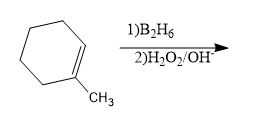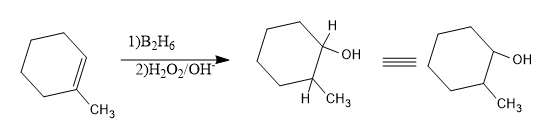# 1-Methylcyclohexane is allowed to react with ${{B}_{2}}{{H}_{6}}$. The product is then treated with ${{H}_{2}}{{O}_{2}}$ and NaOH. The product formed is:A) 1-methyl cyclohexanolB) 2-methyl cyclohexanol ${{B}_{2}}{{H}_{6}}$ C) Methyl cyclohexaneD) CyclohexanolVerified
176.7k+ views
Hint:. The reaction involved is the hydroboration – oxidation reaction.
- The nucleophile added in this reaction is $O{{H}^{-}}$.

Here 1-methylcyclohexane reacts with ${{B}_{2}}{{H}_{6}}$ and the obtained product is treated with ${{H}_{2}}{{O}_{2}}$ and NaOH, we have to predict the product obtained.
- Here, we know that while the reactant, 1-methyl cyclohexane when reacts with diborane, hydroboration reaction takes place and the obtained product undergoes oxidation due to the reaction with ${{H}_{2}}{{O}_{2}}$ and NaOH.
- Hydroboration- oxidation reaction is the reaction in which an alkene undergoes a reaction with diborane and which is oxidized to give alcohol.
So simply we can say that alkene will be converted to alcohol during hydroboration –oxidation.
- In hydroboration –oxidation reaction product will be formed according to the anti-Markovnikov's rule i.e. the nucleophile will attack the reactant in the position of carbon having higher number of hydrogen or can be said as the nucleophile attack the least substituted carbon.

Now let’s see this reaction.
- First we should know the nucleophile and electrophile attacking. By the process of hydrolysis $O{{H}^{-}}$ and ${{H}^{+}}$ ions are formed. So${{H}^{+}}$ is the electrophile and $O{{H}^{-}}$ is the nucleophile formed.

Now where do the nucleophile attacks?
- We know that the nucleophile will attack the least substituted C or C with more hydrogen. Let’s check the number of H across the carbons with double bonds.
- The C attached to the methyl group ($-C{{H}_{3}}$) is having no H, since the valency of C is 4 and the other C is having one hydrogen. So the nucleophile will attack that carbon.
- And the electrophile i.e. ${{H}^{+}}$ will attach with the methyl group substituted C.
Hence the product will be:Now let’s write the name of the product obtained.
- In the obtained cyclic structure, numbering should start from the C attached to –OH group and a methyl group is attached to the second carbon, hence the name will be 2-methyl cyclohexanol.
So, the correct answer is “Option B”.

Note: We should know whether the product is formed through Markonikov’s rule or through Anti-Markovnikov's rule.
- In the naming of the product cyclohexanol is given instead of hexanol, since it is a cyclic structure, so a prefix –cyclo should be given while naming. And the –ol comes as the suffix, since the functional group is alcohol.